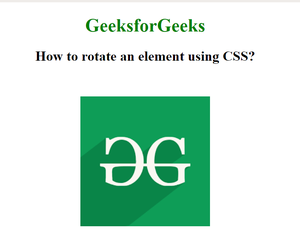GeeksforGeeks App
Open AppBrowser
Continue

# How to rotate an element using CSS ?

The purpose of this article is to rotate an HTML element by using CSS transform property. This property is used to move, rotate, scale and to perform various kinds of transformation of elements.

The rotate() function can be used to rotate any HTML element or used for transformations. It takes one parameter which defines the rotation angle. The rotation angle consists of two parts, the value of the rotation followed by the unit of rotation. The unit can be defined in degrees (deg), gradient (grad), radians (rad) and turns.

Syntax:

`transform: rotate(90deg);`

Example: The following example demonstrates rotation of an image by 45 degree.

## HTML

 `  ` ` `` ``<``html``> `` ` `<``head``>     ``    ``<``style``> ``        ``body { ``            ``text-align:center; ``        ``} ``        ``h1 { ``            ``color:green; ``        ``} ``        ``.rotate_image { ``            ``transform: rotate(45deg); ``        ``} ``    `` `` `` ` `<``body``> ``    ``<``h1``>GeeksforGeeks ``    ``<``h2``>``        ``How to rotate an element using CSS?``    `` ``     ` `     ``<``img` `class``=``"rotate_image"` `src``= ``"https://media.geeksforgeeks.org/wp-content/cdn-uploads/20190710102234/download3.png"``          ``alt``=``"GeeksforGeeks logo"``> `` `` ` ``

Output:
Before Rotation:After Rotation:Supported Browsers: